# 静态代码分析神器 - 数据流分析

## 什么是数据流分析

• 在编译器中，通过数据流分析，编译器可以了解变量间的定义和使用关系，从而在编译期间提前计算部分常量传播 (Constant Propagation) 优化，降低程序运行时所需的计算量。
• 数据流分析也可以协助编译器检查某段代码是否可达 (在动态运行时能不能执行到)，编译器可以结合分析结果，移除部分不可达的代码
• 在软件开发和测试中，数据流分析作为静态分析方法，在程序不运行的情况下，对变量的声明、赋值、使用进行分析，并形成合理代码的改进建议

## 什么是基本块

int x = 1;
int sum = 0;
while( x <= 100)
{
sum += x;
x ++;
}
printf ("%d\n", sum);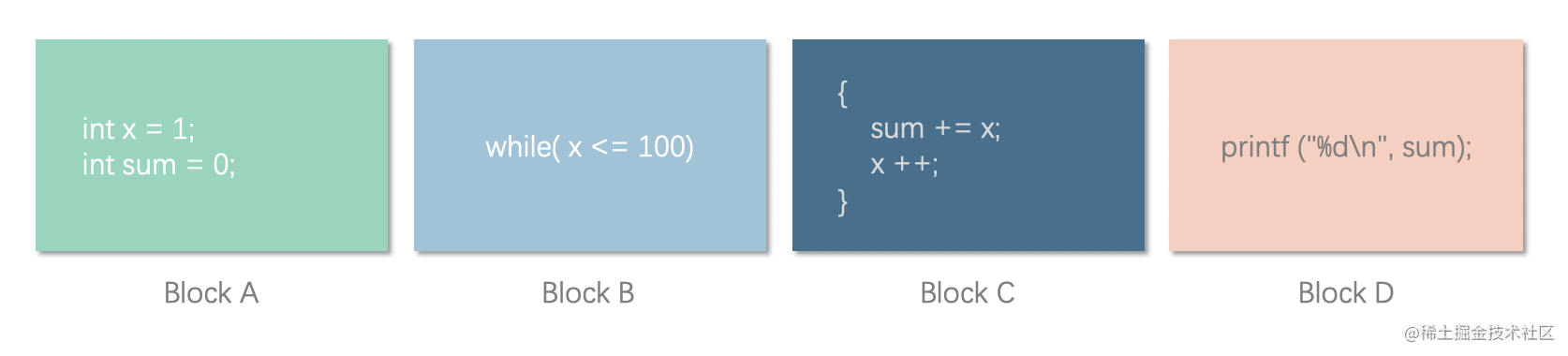(dec,    x,     )
(assign, x,   1 )
(dec,    sum,   )
(assign, sum, 0 )
L0:
(jump if not, x <= 100, L1)
(+=,   sum, x)
(+=,   x  , 1)
(jump, L0 ,  )
L1:
[...]
(call, printf, )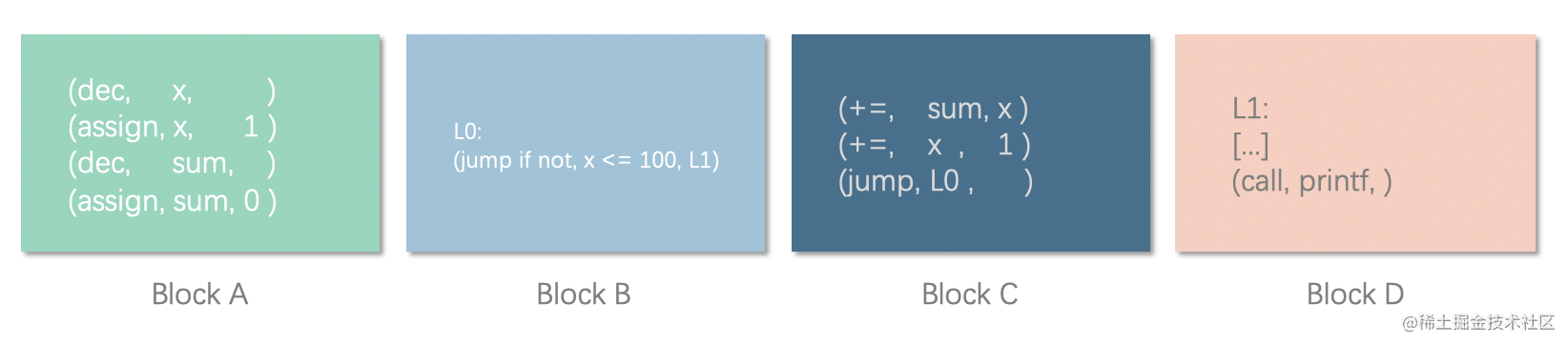## 从基本块到控制流图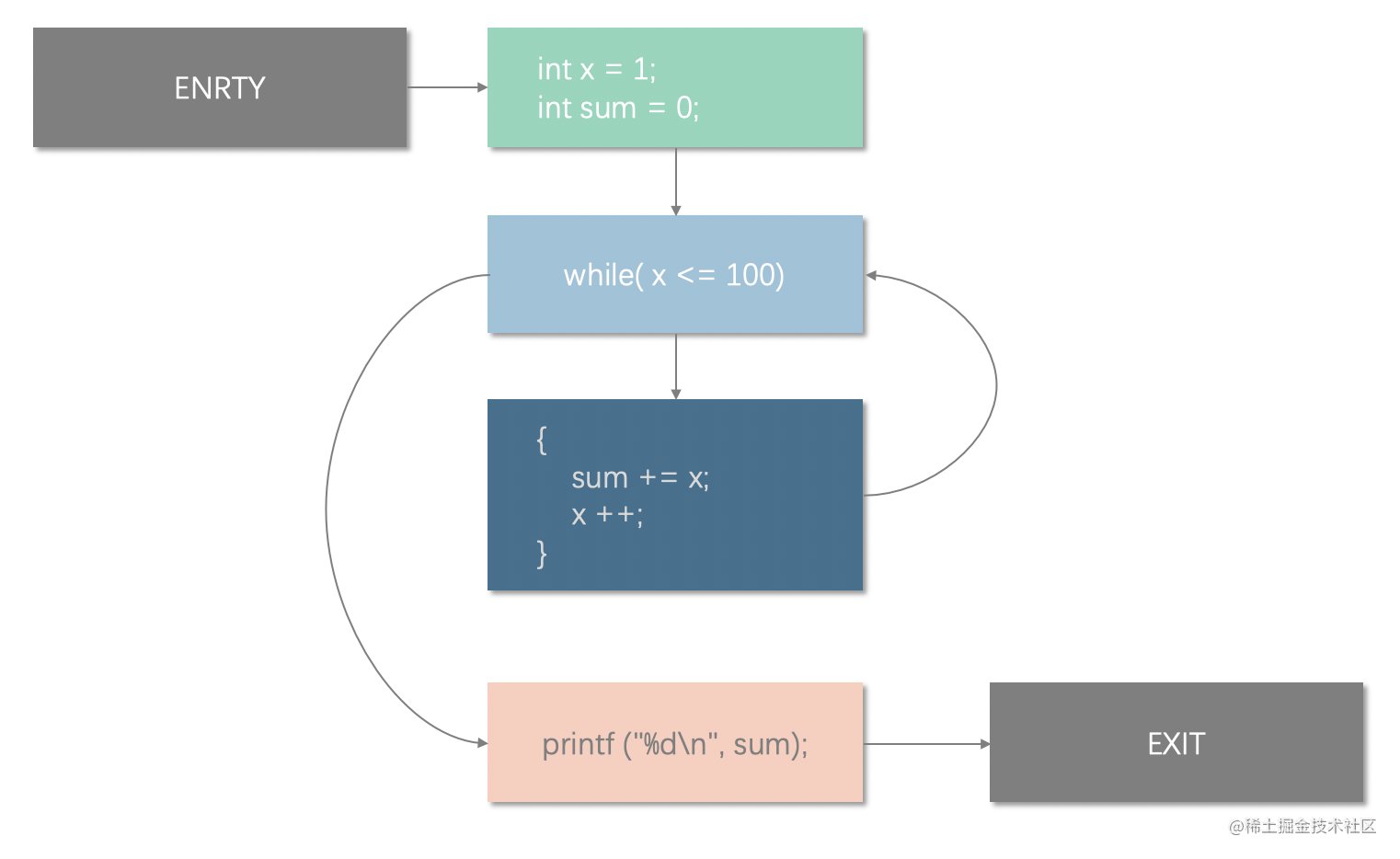## 定值？？使用？？？

• 定值 (definition, def): 一般指变量的赋值，一般包括变量声明和初始化、复制、形式参数列表等
• 使用 (use): 使用变量的值进行运算，一般包括表达式、逻辑判断条件，把变量作为参数传递，返回值等

int a = 20;
int b = a + 10;

$def = \{ a, b\} \\ use = \{ a \}$

## 变量的 “生成” 和 “被杀死”

• 生成 (Genenrate, gen) - 定值的生成，也就是变量的定义、初始化
• 杀死 (Kill) - 路径上的前驱节点以及给出的定值在本语句中被改写，即前驱节点生成的定值被 “杀” 掉了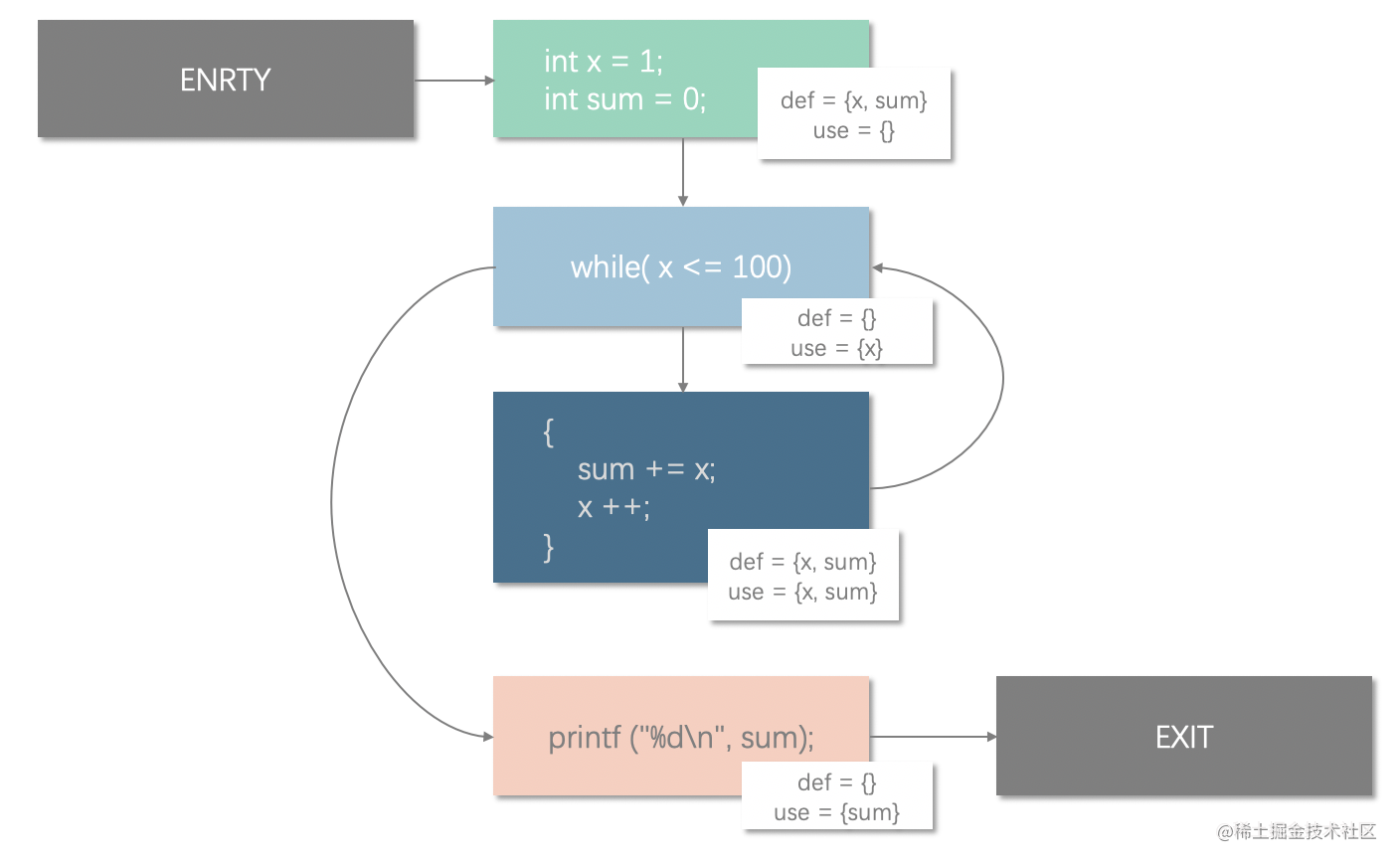$Gen[A] = \{x, sum\}$

$Kill[C] = \{x, sum\}$

## 数据流分析的方式

• $IN[B_{i}]$ 为路径上前驱节点 $B_i$ 发出的到达当前节点的定值集合
• $OUT[B_{j}]$ 为当前节点向路径上的后继节点 $B_j$ 发出的最新定值集合

$\bigcup_{i}^{0..n}IN[i]$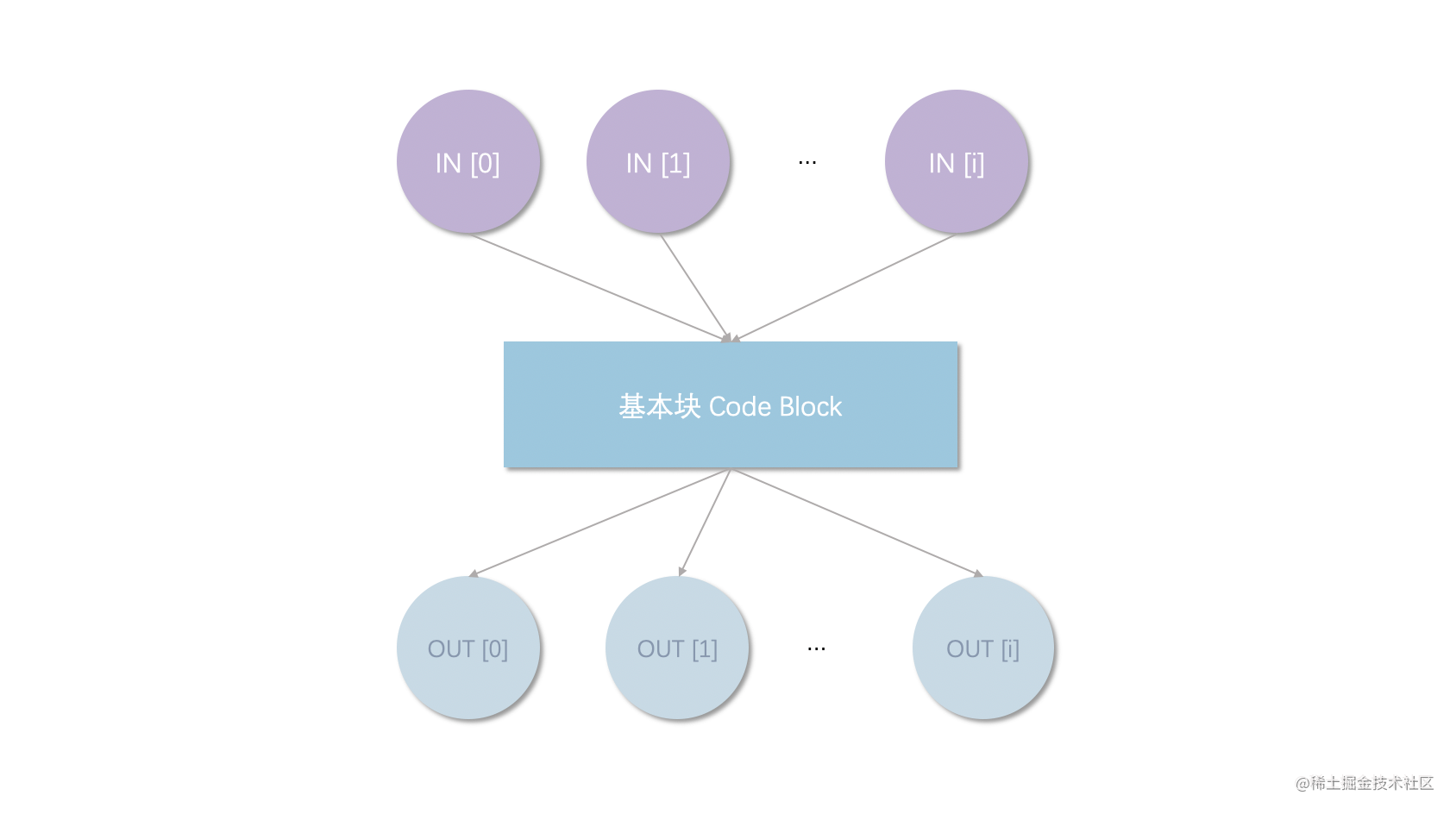$OUT[B] = f_s(\bigcup_{i}^{0..n}IN[i])$

$IN[B] = f_s(\bigcup_{i}^{0..n}OUT[i])$

$IN[ENTRY] = \emptyset$
$OUT[EXIT] = IN[EXIT]$

## 到达定值$\bigcup_{i}^{0..n}IN[i] = IN \cup IN \cup ... \cup IN[n]$

$f_s(B_i) = (\bigcup_{i}^{0..n}IN[i] - Kill[B_{i}])\cup Gen[B_{i}]$

KillGenerate
Kill[A] = $\emptyset$;Gen[A] = $\{x_{a}, sum_{a}\}$
Kill[B] = $\emptyset$;Gen[B] = $\emptyset$
Kill[C] = $\{x_{a}, sum_{a}, x_{c}, sum_{c}\}$;Gen[C] = $\{x_{c}, sum_{c}\}$
Kill[D] = $\emptyset$;Gen[D] = $\emptyset$

BlockINOUT
ENTRY$\emptyset$$\emptyset$
A$\emptyset$$\{x_{a}, sum_{a}\}$
B$\{x_{a}, sum_{a}, x_{c}, sum_{c}\}$$\{x_{a}, sum_{a}, x_{c}, sum_{c}\}$
C$\{x_{a}, sum_{a}, x_{c}, sum_{c}\}$$\{x_{c}, sum_{c}\}$
D$\{x_{a}, sum_{a}, x_{c}, sum_{c}\}$$\{x_{a}, sum_{a}, x_{c}, sum_{c}\}$
EXIT$\{x_{a}, sum_{a}, x_{c}, sum_{c}\}$$\{x_{a}, sum_{a}, x_{c}, sum_{c}\}$